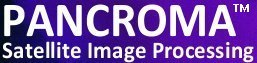See additional satellite image processing articles at www.PANCROMA.com menu selection 'White Papers'

# FFT Image Analysis and Graphical FFT Filtering

Introduction

Fourier Analysis has many applications to the field of satellite image processing. The idea behind Fourier analysis is as follows: a function of time f(t), can be approximated by a linear combination of harmonic functions (sines and cosines) of increasing frequencies, specifically, multiples of a fundamental harmonic of f(t). If you know the right (linear) contributions of all frequencies (Fourier coefficients), that knowledge is equivalent to knowing the function. Put another way, a time dependent function f(t) can be transformed and written equivalently as a frequency-dependent function F(ω). The frequency-dependent function (the spectrum) expresses the frequencies (linear combination of multiples of a fundamental frequency) and the magnitudes of each of those frequencies. The Fourier Transform is a mathematical equation that computes the coefficients that, multiplied by cosine and sine functions expresses f(t) in terms of F(ω). The amazing part is that this is true regardless of the complexity of f(t), and works even if f(t) is not periodic!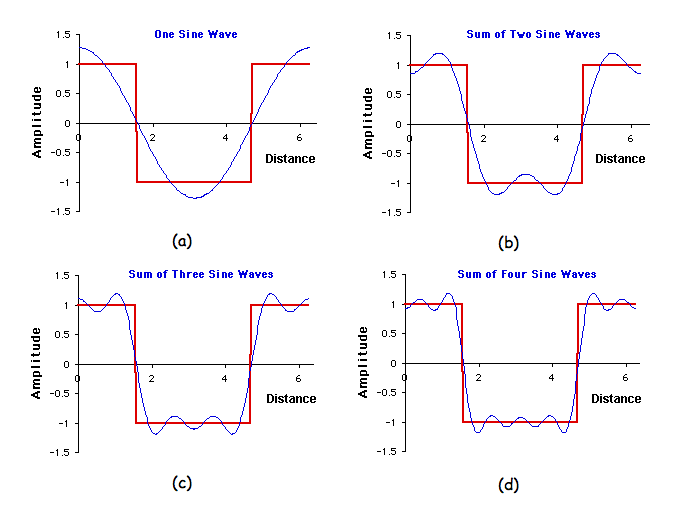The square wave can be approximated by the linear combinatation of four sine waves.

One key to the utility of the Fourier Transform is that it has an inverse, which is very similar to the forward transform mathematically, except that it transforms F(ω) back into f(t). Both transformations are linear, so that, if a and b are any constants, the transformations take a*f(t) + b*g(t) into a*F(ω) + b*G(ω) and back again. Another key is that it is not necessary to include all of the harmonics in the frequency-dependent function to approximate the time-dependent function. The more that are included, the closer the approximation will be, but in many cases only a few harmonics need be included to adequatly describe f(t).

Intuitively, it is best to think of the Fourier transformation as approximating a function by sines and cosines. However, the actual formulas become much simpler and more general if the transformation is re-cast as an approximation by a sum of complex exponentials according to Euler's equation:

exp(ix)= cos(x) + isin(x)

In this new expression, which is called the complex Fourier transformation, the transformed function f may be complex, and its transformation F is alwyas a complex function. All the basic properties (the inverse, linearity, convolution etc.) remain the same, as expressing the Fourier transformation in complex notation is mathematically equivalent to the more cumbersome sine and cosine version.

In image processing, the image is considered to only have a real component, i.e. the imaginary component is always zero. However, the Fourier transform of an image resides in the complex plane and has both types of components.

One property of the Fourier transformation that is very useful in graphics and imaging is known as the convolution theorem. Technically, a convolution of two functions is an integral of their product, where one function is displaced relative to the other. For example a filter is normally convoluted with an image in the neighborhood of a pixel. In order to filter the entire image, one must slide the filter around and convolve it with the image around every pixel.

The convolution theorem states that the Fourier transformation of the convolution integral is the product of the transforms of the two functions. That means that we can transform the image, and the filter multiply the two, and inverse-transform the product. The result is the filtered image, without the need to multiply and sum around every pixel. The price that we pay for this simplification is in transforming the functions back and forth, but with a fast Fourier algorithm this technique can save a great deal of computational time for large convolution kernels. There are many good descriptions of the Fourier transformation in the literature.

The method for calculating the Fourier transform of an image is to take the one-dimensional FFT of each of the rows, followed by the one-dimensional FFT of each of the columns. Specifically, start by taking the FFT of the N pixel values in row 0 of the real array. The real part of the FFT's output is placed back into row 0 of the real array, while the imaginary part of the FFT's output is placed into row 0 of the imaginary array. After repeating this procedure on rows 1 through N-1, both the real and imaginary arrays contain an intermediate image. Implementing a convolution using Fourier transforms is generally complex, as the forward and reverse transforms can "wrap" and contaminate the leading frequency bins, requiring that the images be padded with zeros or image buffers. The convolution kernal requires careful handling as a result of the characteristics of the frequency space.

Next, the procedure is repeated on each of the columns of the intermediate data. Take the N pixel values from column 0 of the real array, and the N pixel values from column 0 of the imaginary array, and calculate the FFT. The real part of the FFT's output is placed back into column 0 of the real array, while the imaginary part of the FFT's output is placed back into column 0 of the imaginary array. After this is repeated on columns 1 through N-1, both arrays have been overwritten with the image's frequency spectrum.

The image transform is usually expressed as the magnitude of the vector consisting of real and complex (orthogonal) components. The quadrants are also swapped so that zero frequency appears in the middle of the image, rather than at coordinate [0,0]. The following figures show graphically how the spatial data and frequency data are stored. Those not familiar with the Fourier transform may be surprised to see that the frequency domain contains both positive and negative frequencies. The sign of a frequency can be thought of as indicating the direction of rotation of a vector on the complex plane. (Negative frequencies are redundant for real spatial domain data, as a result of the symmetry of the Fourier transform for this class of data. Never the less, they must be handled as they are computed by the FFT algorithm).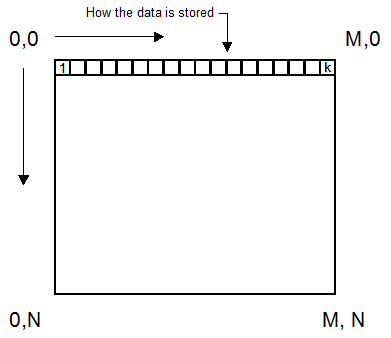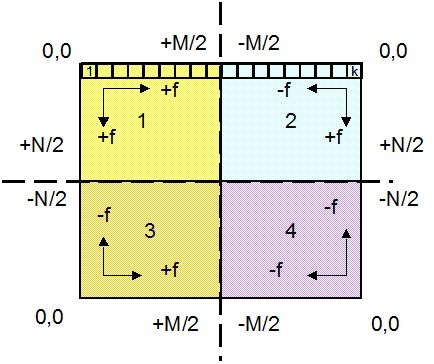2D image in the spatial domain and its Fourier transform into the frequency domain. The data is typically stored in a 1D array as shown.

After the quadrants are swapped, the origin of the transformed image is at the center.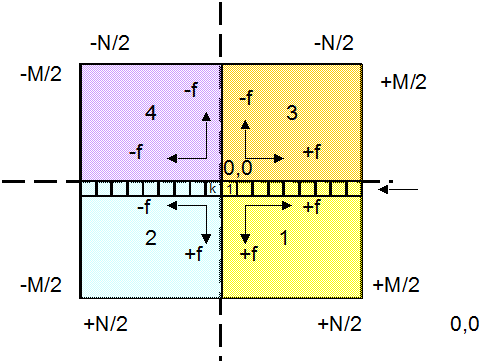The quadrants are usually swapped so that the low frequencies are at the center. Note that there are only N/2 by M/2 degrees of freedom in the transformed image. This is usually explained in terms of the Nyquist equation, which states that the highest frequency that N pixels can express is N/2.

One Way FFT

IMPORTANT NOTE: The FFT used by PANCROMA™ is very fast computationally, but it needs a lot of memory. Moreover, it needs at least single precision (32 bit) FLOAT types to compute forward and reverse transforms. As a result, you must watch your memory resources carefully. PANCROMA will be able to process full-size Landsat multispectral bands on most modern systems, but is not able at this time to process the panchromatic band.

In order to compute the frequency spectrum of an image, first open a grayscale image. Then select 'FFT' | 'Image Fourier Analysis' | 'Forward 2D Image Transform'. A dialog box will appear allowing you to input a scale factor. Because the spectra are often fractional values less than one, it is usually necessary to scale them for display. The default value is 1000.

The FFT output consists of a number real and an imaginary part. PANCROMA will display the magnitude of the vector consisting of these parts, according to the equation: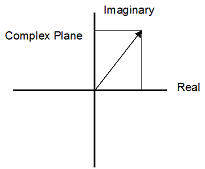Complex plane, showing the magnitude vector graphically.

Some examples of the magnitude frequency spectra of some images are shown below: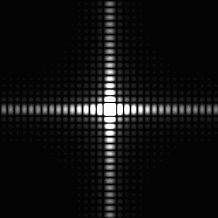A 2D square pulse and its transform

Note that Fourier transform for the square is analogous to the corresponding transform for a square pulse shown below.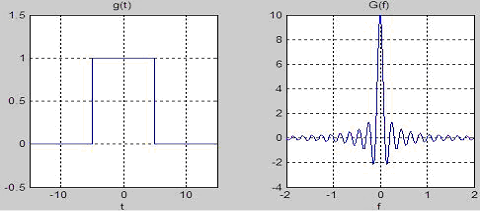A 1D square pulse.

Important features of the Fourier transform is that the maximum frequency is half of the discrete spatial dimensions. That is, if the column dimension is M, it is not possible for that dimension to support a frequency higher than M/2 (which would correspond to alternation in every other image pixel). The quantity M/2 is called the Nyquist frequency. Another important feature is that the Fourier transform, unlike the spatial function has both positive and negative components.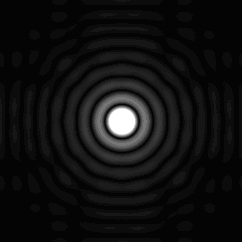A 2D cylindrical pulse and its transform.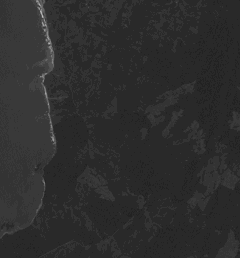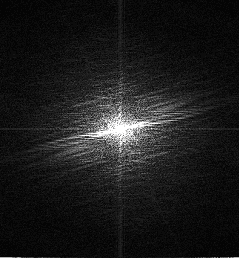A Landsat multispectral band and its transform.

2D Graphical Frequency Filter

PANCROMA™ has a utility that takes advantage of features of Fourier transformed images that let you to graphically design your own frequency spectrum filters. One of the great advantages of transforming images from the spatial domain to the frequency domain is the ease of designing filters.

In order to filter an image, select 'File' | 'Open' and open a single grayscale image. Now select 'FFT' | 'Image Fourier Analysis' | '2D Image Fourier Graphical Filter' | 'Magnitude Filter'. A dialog box will again appear allowing you to input a scale factor. Because the spectra are often fractional values less than one, it is usually necessary to scale them for display. The default value is 1000.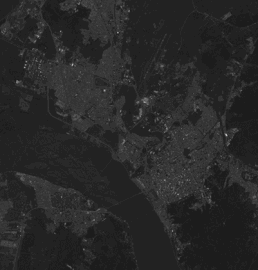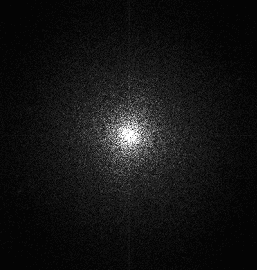A Landsat multispectral band and its transform.

When the transformed image becomes visible you will be able to mark it using you cursor. The top menu allows you to select your pen width and select your masking shapes. Using your cursor, mark out any frequencies that you want to remove from your image. For example, if we wished to remove all high frequencies from the image, we would retain the center of the transformed image and remove the outside frequencies (low frequencies are in the middle).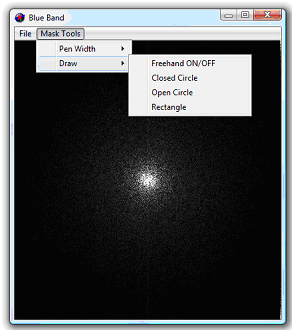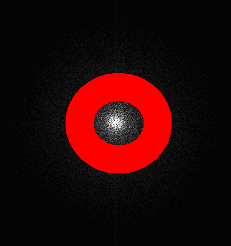At the left is the menu showing the masking tools. At the right is a low pass filter, established graphically.

The idea behind the mask tools is to remove frequencies from the image. Any frequency covered by red will be eliminated from the Fourier Transform of the image, that is, both the real and imaginary components of the transformed image will be zeroed. Another way of saying this is that the masked area will be convolved with the image. In this case the function H[u, v] consisting of all ones outside the mask and all zeros in the mask area will be multiplied by the Fourier Transform of the image. Then the product will be transformed back to the spatial domain.

In order to use the mask shapes, click on the upper left and lower right corners, then choose the menu item. (This applies to all shapes: rectangle, closed circle (ellipse), open circle). When you are done defining your filter select 'Exit' from the menu. The designated frequencies will be removed from the transform and the result will be inverse transformed back to the spatial domain. The result of this example is shown below.

IMPORTANT NOTE: Because of the severe RAM requirements, no 'Undo' function is available. If you decide that you do not like your mask, you will have to start over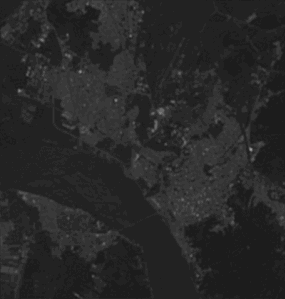The filtered image. Significant smoothing is apparent.

Considerable smoothing has occurred in the image as a lot of high frequency information has been removed. You can perform other useful filtering operations using this technique.

In order to filter the low frequencies and pass the high, use a solid circle centered on (0, 0). You can build many other useful and interesting frequency domain filters by studying your image spectra and experimenting with masks.

This paper has introduced FFT image processing, demonstrating its use in creating filters. However the real power of FFT image processing is image deconvolution, which is described in the next paper in this series.